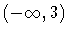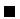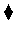Next: A Project Management Problem Up: Adding Computation Domains Previous: Non-Linear Solver: Intervals

Subsections

# Some Useful Primitives

Besides those already mentioned, it is usual that additional primitives are provided in CLP systems. We will mention some primitives which are available in Prolog IV; the reader is advised to refer to the manual of the CLP system being used.

## The Bounds of a Variable

Sometimes accessing the bounds of a variable is useful. Could this be made using the interval constructors to access the bounds of the variables?

?- A ~ co(1, 3), A ~ co(L, U).
U ~ gt(1),
L ~ lt(3),
A ~ co(1,3).


This does not work as expected: the lower and upper bounds are not returned as simple numbers, but rather as (infinite) sets of numbers. Additionally, the intervals might not be as desired if we do not access the variable using the same combination of open/closed interval it has at that moment:

?- A ~ co(1, 3), A ~ oc(L, U).
U ~ ge(1),
L ~ lt(3),
A ~ co(1,3).


What happens here is that we are not accessing the bounds of the variable A, but rather constraining the variables L and U. When L is constrained to be a lower bound of the interval [1, 3), then L can take any value from 3 (excluded) downwards (i.e., the range).

There are specialized primitives which access the greatest lower bound of an interval variable (glb(A, L)), the lowest upper bound (lub(A, U)) and both at the same time: bounds(A, L, U):

?- A ~ co(1, 3), glb(A, L).
L = 1, A ~ co(1,3).

?- A ~ co(1, 3), lub(A, U).
U = 3, A ~ co(1,3).

?- A ~ co(1, 3), bounds(A, L, U).
U = 3, L = 1, A ~ co(1,3).


This can be used, in certain cases, to force a maximization/minimization of the solution of a problem:

?- X = A .-. B, A ~ cc(1,3), B ~ cc(3,7), glb(X,X).
B = 7, A = 1, X = -6.


## Enumerating Variables

Very commonly the problem constraints do not suffice to give definite values to the variables. In this case obtaining solutions must be made resorting to an enumeration of the remaining values in the domain of each (or some) variables. With finite domain variables this enumeration poses no problem other than performance, since the number of possible values in the domain is finite. With interval variables the situation is more awkward, because the set of points in the interval of the variable is, in principle, infinite. To help in this case, the primitive enum/1 instantiates its argument, which must be an interval variable, to the integer values in its domain.

Example 3.3   This piece of code decomposes a number (1001, in this case) into two factors:

?- Num = 1001, Num = A .*. B, A ~ cc(1,Num), B ~ (1,A),
enum(B), enum(A).
B =  1, A = 1001, Num = 1001;
B =  7, A =  143, Num = 1001;
B = 11, A =   91, Num = 1001;
B = 13, A =   77, Num = 1001.


The key point for the solution is the enumeration: it generates integer numbers in the domain of the variables, which are automatically tested against the constraints. If those numbers are not generated, the system does not have any way of factoring Num:

?- Num = 1001, Num = A .*. B, A ~ cc(1,Num), B ~ cc(1,A).
Num = 1001, B  ~  cc(1,1001), A  ~  cc(1,1001).Problem 3.9   Use the code in Example 3.3 to factor bigger numbers. Try interchanging the order of the enumeration. Is there any difference? Why?Prolog IV provides a number of enumeration and splitting primitives, which are useful in a variety of contexts.Next: A Project Management Problem Up: Adding Computation Domains Previous: Non-Linear Solver: Intervals
MCL
1998-12-03